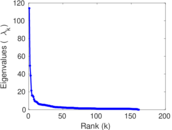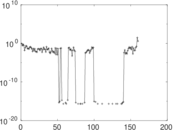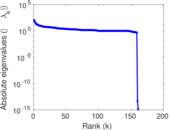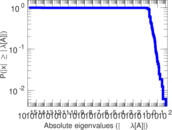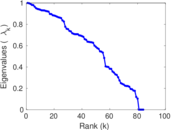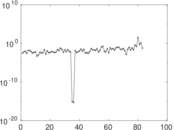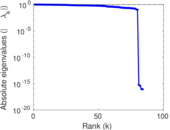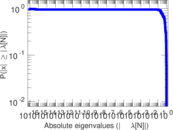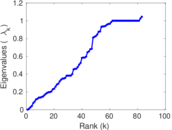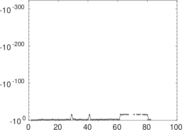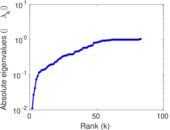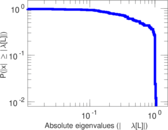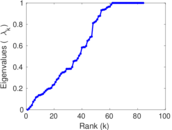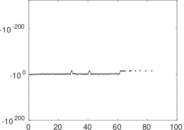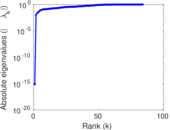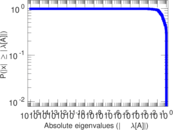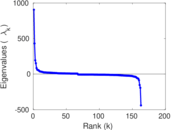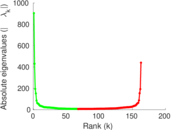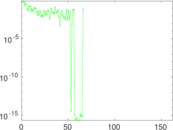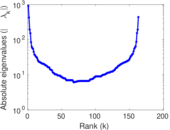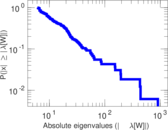# Wiktionary edits (sg)

This is the bipartite edit network of the Sango Wiktionary. It contains users and pages from the Sango Wiktionary, connected by edit events. Each edge represents an edit. The dataset includes the timestamp of each edit.

 Code `msg` Internal name `edit-sgwiktionary` Name Wiktionary edits (sg) Data source http://dumps.wikimedia.org/ AvailabilityDataset is available for download Consistency checkDataset passed all tests Category Authorship network Dataset timestamp 2017-10-20 Node meaning User, article Edge meaning Edit Network formatBipartite, undirected Edge typeUnweighted, multiple edges Temporal dataEdges are annotated with timestamps

## Statistics

 Size n = 909 Left size n1 = 163 Right size n2 = 746 Volume m = 3,094 Unique edge count m̿ = 1,555 Wedge count s = 64,563 Claw count z = 2,728,856 Cross count x = 104,488,505 Square count q = 48,601 4-Tour count T4 = 650,478 Maximum degree dmax = 878 Maximum left degree d1max = 878 Maximum right degree d2max = 45 Average degree d = 6.807 48 Average left degree d1 = 18.981 6 Average right degree d2 = 4.147 45 Fill p = 0.012 788 0 Average edge multiplicity m̃ = 1.989 71 Size of LCC N = 612 Diameter δ = 13 50-Percentile effective diameter δ0.5 = 4.943 18 90-Percentile effective diameter δ0.9 = 7.503 47 Median distance δM = 5 Mean distance δm = 4.965 46 Gini coefficient G = 0.747 724 Balanced inequality ratio P = 0.197 641 Left balanced inequality ratio P1 = 0.125 404 Right balanced inequality ratio P2 = 0.234 971 Relative edge distribution entropy Her = 0.827 205 Power law exponent γ = 2.908 24 Tail power law exponent γt = 2.051 00 Tail power law exponent with p γ3 = 2.051 00 p-value p = 0.003 000 00 Left tail power law exponent with p γ3,1 = 1.711 00 Left p-value p1 = 0.557 000 Right tail power law exponent with p γ3,2 = 2.191 00 Right p-value p2 = 0.000 00 Degree assortativity ρ = −7.547 11 × 10−5 Degree assortativity p-value pρ = 0.997 627 Spectral norm α = 114.064 Algebraic connectivity a = 0.011 108 4 Spectral separation |λ1[A] / λ2[A]| = 2.307 92 Controllability C = 586 Relative controllability Cr = 0.648 230

## Plots

### Fruchterman–Reingold graph drawing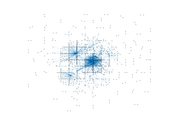### Degree distribution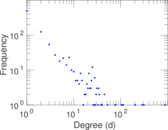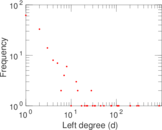### Cumulative degree distribution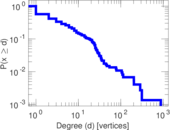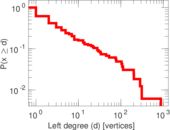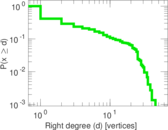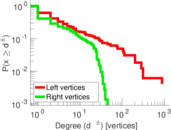### Lorenz curve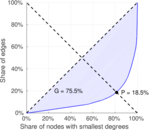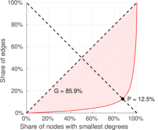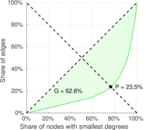### Spectral distribution of the adjacency matrix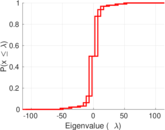### Spectral distribution of the normalized adjacency matrix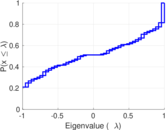### Spectral distribution of the Laplacian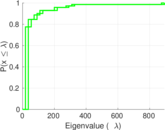### Spectral graph drawing based on the adjacency matrix### Spectral graph drawing based on the Laplacian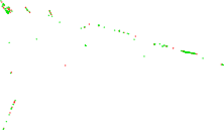### Spectral graph drawing based on the normalized adjacency matrix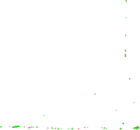### Degree assortativity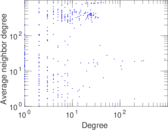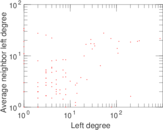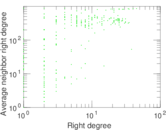### Zipf plot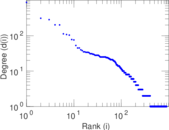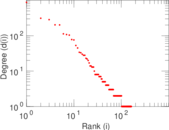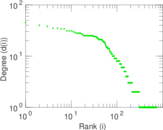### Hop distribution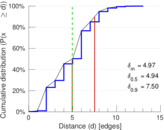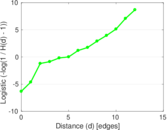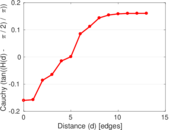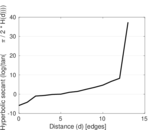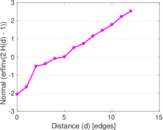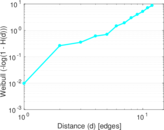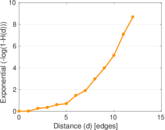### Double Laplacian graph drawing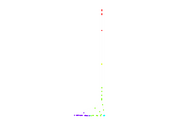### Delaunay graph drawing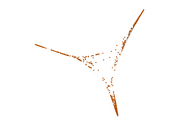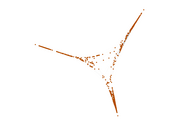### Edge weight/multiplicity distribution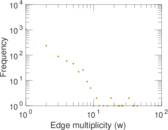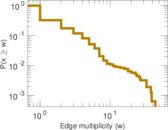### Temporal distribution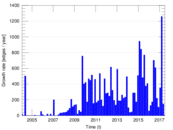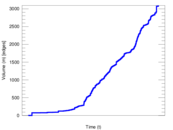### Temporal hop distribution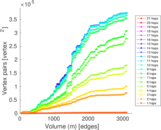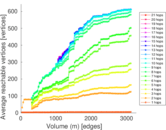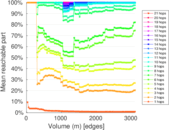### Diameter/density evolution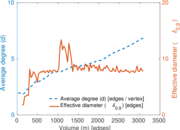### Matrix decompositions plots